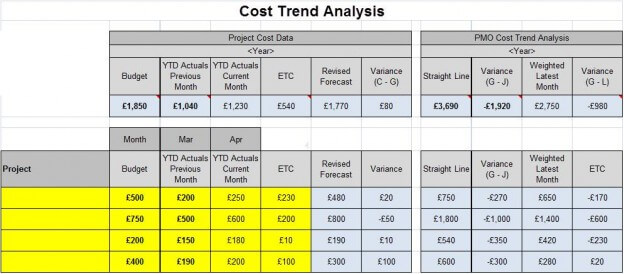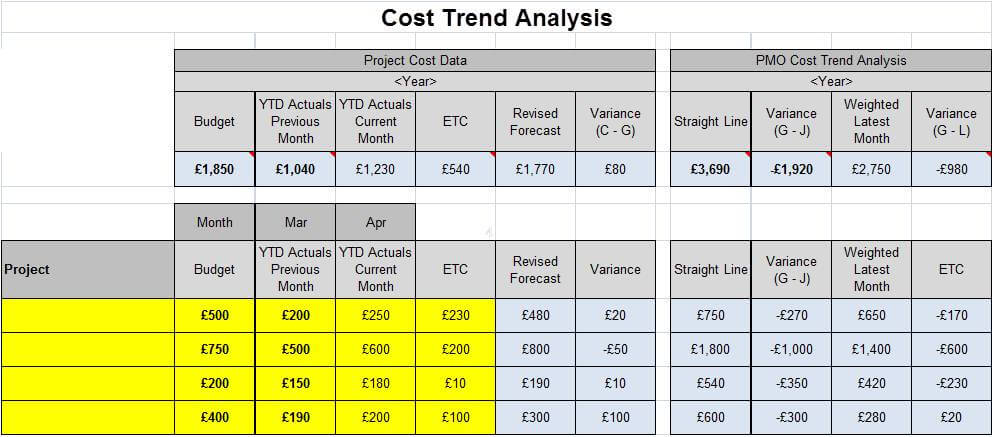# Example of simple PMO cost trend analysis tool

The last post, How a PMO can use cost trend analysis to identify project budget under and overruns, it covered how using cost trend analysis can help a PMO quickly identify where there may be budget issues.  This post will cover a simple approach that can be used to conduct this analysis.

### Objective

The objective of conducting this analysis is to identify where a project will under or overrun budget.  While a project manager should be completing budget reviews and re-forecasts on a regular basis, unfortunately this is not always the case.  This is made worse as there is a reluctance of project managers to give up excess budget “just in case” they need it.

### Data Needed

• List of projects
• Budget for each project
• Previous months Year to Date (YTD) Actuals for each project
• Current months YTD Actuals for each project
• Estimate to complete (ETC) for each project

This data should be produced by the project on a monthly basis so hopefully should not present an issue.

### Straight Line Cost Trend Analysis

This is a very simple approach.  Take the current months YTD Actuals, then calculate the numerical value for the current month:

• January = 1
• February = 2
• March = 3
• December = 12

Divide the current months YTD Actuals by the numerical value for the current month.  So if you were using the YTD Actuals for April, you would divide by 4.  Then multiply the result by 12 to give the annualised forecast. The forecast can then be compared against the original budget or the revised forecast from the project manager. Note: while a simple approach, most projects ramp up monthly spend over time.  Therefore, the approach can give lower predictions where a project has only recently started.  It should work well for stable multi year projects that have small changes in monthly spend.

### Simple Weighted Cost Trend Analysis

This approach addresses the issue where a project will ramp up monthly costs during mobilisation by using the latest months actuals to calculate forecast future spend. To do this, you need the current months actuals.  If this is not available it can be calculated by taking the current months YTD Actuals minus the previous months YTD Actuals. Then calculate the numerical value for the current month (in the same way as used in the Simple Cost Trend Analysis). Calculate the remaining months in the year.  So for April you will calculate 12 minus 4 to give 8. Then calculate current month Actuals (not YTD) multiplied by remaining months in year.  When complete add the current month YTD Actuals.  This will give you a forecast that includes the YTD Actuals and then an estimate weighted on current month actuals.  The logic being that future months are more likely to be similar to the most recent months spend. Note: be careful that latest month actuals is representative and does not include large “one-off’s” i.e. hardware, infrastructure purchasers, etc.  This will distort the numbers.  You may wish to take these out of the monthly calculation and add back at the end.

### Analysis Results

When the results have been calculated, the forecast can then be compared against the latest forecast from the project manager.  This will highlight potential under or overruns. The PMO should then meet with the project manager to understand their forecast and to review if there is a credible plan.  If there is no clear reason to support the forecast from the project, the PMO should encourage for the estimate to be reviewed and updated. This will ensure that overruns are identified early, remediation action to be taken and avoid budget shocks to the sponsor.  If there are significant increases, make sure the business case still makes sense and if not, consider stopping the project. If the review shows an under run, push the project to release funds so that they can be used to fund other opportunities.

### Example

Below is an example of a Cost Trend Analysis template that calculates both the straight line and weighted forecasts. This template is available as a free download.  Simply click on the link below the picture to download.Click to download PMO Cost Trend Analysis Template